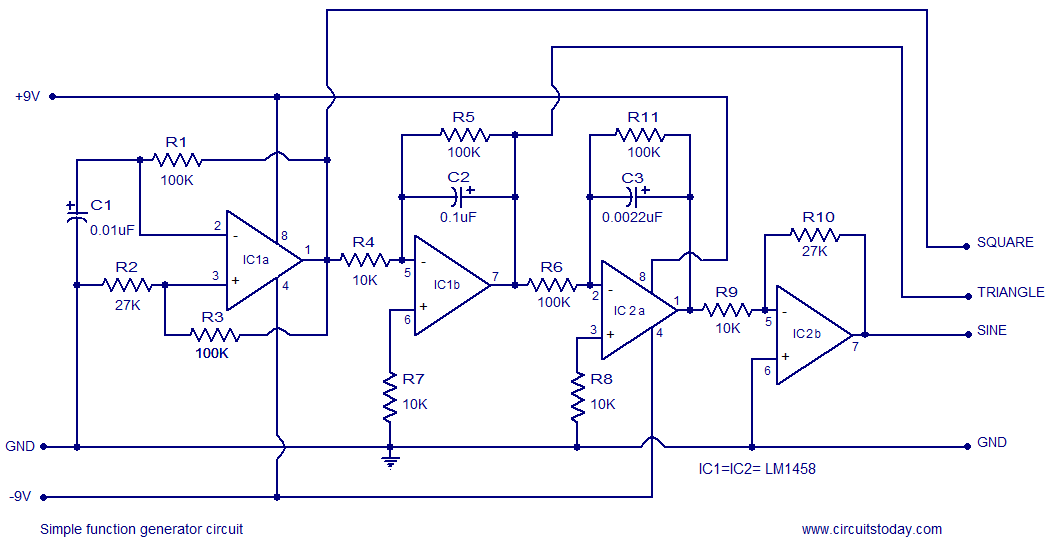## Audio

[Audio][slideshow]

## LM1458 Simple Function Generator.

A simple function generator circuit using LM1458 is known here. LM1458 is a dual general purpose operational amplifier. The two opamps inside LM1458 has a common bias network, power supply line and are independent of each other in operation. The LM1458 does not require an external frequency compensation circuit and has built in short circuit protection. Lm 1458 has a wide supply voltage range and it is available in 8 pin mini DIP package.

### Description.

Four opamps (2 from each IC) is used in the function generator circuit. First opamp IC 1a is wired as an astable multivibrator.R1 is the feedback resistor and C1 is the timing capacitor output of IC 1a is feed back to its non inverting input (pin 3) from the junction of R3 & R2.The output of IC 1a will be a square wave and its frequency can be varied by varying R1 or C1.

The next opamp IC 1b is wired as an integrator.R5 is the feedback resistor and C2 is the integrating capacitor. Non inverting input of IC 1b (pin6) is tied to ground using resistor R7. The output of IC 1a which is a square wave is applied to the inverting input of IC 1b (pin 5) through R4 which is the input resistance of IC 1b.The output of IC 1b will be a triangular wave form, because integrating a square wave will result in a triangular waveform.

IC 2a forms another integrator, where R11 is its feedback resistor and C3 is the integrating capacitor.R6 is the input resistance of IC 2a. Non inverting input of IC 2a (pin 3) is tied to ground using the 10K resistor R8. IC 2b forms an inverting amplifier where R9 is its input resistor and R10 the feedback resistor. With the used values of R10 & R9, the gain of the inverting amplifier stage will be 27, (AV = -Rf/Rin). The triangular output waveform from the IC 1b is further integrated using IC 2a inverter using IC 2b circuit diagram.

### Circuit diagram.Simple function generator circuit

### Notes.

• Use +/- 9V dual supply for powering th e circuit.
• Mount IC1 & IC2 on holders.
• The power supply must be well regulated and ripple free.
• The circuit is just an elementary one and is not suitable for high end application where noise, harmonics etc. present in the function generator output would affect the application adversely.

[Lights][grids]